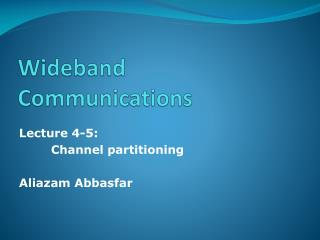Download PresentationWideband Communications

# Wideband Communications - PowerPoint PPT PresentationDownload Presentation## Wideband Communications

- - - - - - - - - - - - - - - - - - - - - - - - - - - E N D - - - - - - - - - - - - - - - - - - - - - - - - - - -
##### Presentation Transcript

1. Wideband Communications Lecture 4-5: Channel partitioning Aliazam Abbasfar

2. Outline • Channel partitioning

3. How to partition channels? • Partition the signal into N dimensions (sub-channels) • Signal duration : T • Channel Bandwidth/Signal energy limits N • Continuous transmission • Received signal

4. ISI and ICI • ICI : Inter-Channel Interference • The interference between sub-channels • Intra Symbol Interference • Orthogonal sub-channels = zero ICI • ISI : Inter Symbol Interference • The interference between subsequent symbols (duration T) • Generalize Nyquist criterion: • qn,m(kT) = d(n-m,k) • Q(k) = d(k) I

5. Modal Modulation • Optimum transmit basis • Eigen-functions of channel autocorrelation function • r(t) = h(t)*h*(-t) • fn(t) * r(t) = rnfn(t) • Orthonormal set exists • The time limit avoids ISI • The noise samples at MFs are independent • E[ninj] = ri N0/2 di,j • SNRn = (rn)2 En /rn N0/2 = rn En / (N0/2) • gn = rn / (N0/2)

6. Modal Modulation (2) • Each eigen-function is a mode (Modal modulation) • gn = rn /(N0/2) • Operate in N best modes (N largest eigen-values) • Each mode is detected independently (best performance) • Optimality • Eigen-functions maximizes the bit rate for N dimensions (for a given set of {En}) • En is computed by water-filling algorithm • Best average SNR for any N orthonormal basis • Disadvantages: • Channel dependent • Difficult to solve • except for no ISI channels : r(kT) = d(k) • Can be found using sampling

7. Other transmit basis • Time-division partitioning • Channel causes both ICI and ISI • ICI and ISI is removed by equalization • ISI can be removed by Guard Time • Frequency division partitioning (MT) • No ICI when no overlap in frequency • No ISI if sub-channel BW is small • Practical filters = Excess bandwidth • MT is MM when T is very large • Best energy allocation

8. OFDM • Orthogonal frequency division multiplexing • fn(t) = rect(t/T) ej2pnf0 t • f0 = 1/T • Guard time removes ISI and ICI • Spectrum • Sinc functions • Cyclic prefix • Periodic channel • Requires multiple OSCs with precise frequency • Analog implementation is hard • Residual ICI add up

9. Discrete channel partitioning • We look at the discrete-time representation of the system • Channel response (p(t)) is sampled at 2x the highest frequency to be used • A vector of (N+v) samples is considered a symbol • T= (N+v) T’ • Each sample can be one dimension (N+v dimensions) • Guard period of v samples is considered for no ISI • Channel response span of time : TH = (v+1)T’ • Discrete basis functions: • fn(t) = Smk,nf(t-kT’) • mn is a (N+v)-dimension vector • p(t) = f(t) * h(t) * f*(-t) • y = P x + n

10. Vector coding • Singular value decomposition • P = F [L | 0 N, n] M* • M is an (N+v)x(N+v) unitary matrix • F is an NxN unitary matrix • L is an NxN diagonal matrix with singular values ln • Vector coding • Choose the first N column of M as transmit basis • Choose N column of F to get x • Discrete matched filters • x = M [X | 0 … 0]T = M [XN-1 … X1 X0 | 0 … 0]T = SXnmn • y = P x + n = F L X + n • Y = F* y = L X + N • Yn = lnXn + Nn • Noises are independent with the same variance • Colored noise • E[nn*] = Rnn = L L* • Whitening • y’ = L-1/2 y = L-1/2 P x + L-1/2 n = F’ L X + n’ • Y’ = F’* y’ = F’* L-1/2 y = L X + N’

11. Vector coding (2) • SNRn for sub-channels • gn = |ln|2 /(N0/2) • Water-filling to allocate energy • Note that there are (N+v) dimensions • Bit rate :

12. Reading • Cioffi Ch. 4.4-5